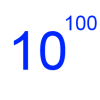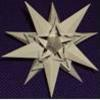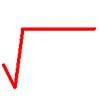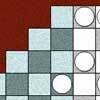Search by Topic

Resources tagged with Powers & roots similar to Fibonacci Fashion:

Filter by: Content type:
Age range:
Challenge level:

There are 37 results

Broad Topics > Numbers and the Number System > Powers & rootsFibonacci Fashion

Age 16 to 18 Challenge Level:

What have Fibonacci numbers to do with solutions of the quadratic equation x^2 - x - 1 = 0 ?Plus or Minus

Age 16 to 18 Challenge Level:

Make and prove a conjecture about the value of the product of the Fibonacci numbers $F_{n+1}F_{n-1}$.Pythagorean Fibs

Age 16 to 18 Challenge Level:

What have Fibonacci numbers got to do with Pythagorean triples?Root to Poly

Age 14 to 16 Challenge Level:

Find the polynomial p(x) with integer coefficients such that one solution of the equation p(x)=0 is $1+\sqrt 2+\sqrt 3$.Googol

Age 16 to 18 Short Challenge Level:

Find the smallest value for which a particular sequence is greater than a googol.Ab Surd Ity

Age 16 to 18 Challenge Level:

Find the value of sqrt(2+sqrt3)-sqrt(2-sqrt3)and then of cuberoot(2+sqrt5)+cuberoot(2-sqrt5).Roots Near 9

Age 14 to 16 Short Challenge Level:

For how many integers 𝑛 is the difference between √𝑛 and 9 is less than 1?Perfectly Square

Age 14 to 16 Challenge Level:

The sums of the squares of three related numbers is also a perfect square - can you explain why?The Root of the Problem

Age 14 to 18 Challenge Level:

Find the sum of this series of surds.Enriching Experience

Age 14 to 16 Challenge Level:

Find the five distinct digits N, R, I, C and H in the following nomogramIn Between

Age 16 to 18 Challenge Level:

Can you find the solution to this algebraic inequality?Consecutive Squares

Age 14 to 16 Challenge Level:

The squares of any 8 consecutive numbers can be arranged into two sets of four numbers with the same sum. True of false?Rational Roots

Age 16 to 18 Challenge Level:

Given that a, b and c are natural numbers show that if sqrt a+sqrt b is rational then it is a natural number. Extend this to 3 variables.Archimedes and Numerical Roots

Age 14 to 16 Challenge Level:

The problem is how did Archimedes calculate the lengths of the sides of the polygons which needed him to be able to calculate square roots?How Many Solutions?

Age 16 to 18 Challenge Level:

Find all the solutions to the this equation.Em'power'ed

Age 16 to 18 Challenge Level:

Find the smallest numbers a, b, and c such that: a^2 = 2b^3 = 3c^5 What can you say about other solutions to this problem?Fit for Photocopying

Age 14 to 16 Challenge Level:

Explore the relationships between different paper sizes.Equal Temperament

Age 14 to 16 Challenge Level:

The scale on a piano does something clever : the ratio (interval) between any adjacent points on the scale is equal. If you play any note, twelve points higher will be exactly an octave on.Archimedes Numerical Roots

Age 16 to 18 Challenge Level:

How did Archimedes calculate the lengths of the sides of the polygons which needed him to be able to calculate square roots?Power Countdown

Age 14 to 16 Challenge Level:

In this twist on the well-known Countdown numbers game, use your knowledge of Powers and Roots to make a target.Unusual Long Division - Square Roots Before Calculators

Age 14 to 16 Challenge Level:

However did we manage before calculators? Is there an efficient way to do a square root if you have to do the work yourself?Staircase

Age 16 to 18 Challenge Level:

Solving the equation x^3 = 3 is easy but what about solving equations with a 'staircase' of powers?Rachel's Problem

Age 14 to 16 Challenge Level:

Is it true that $99^n$ has 2n digits and $999^n$ has 3n digits? Investigate!Lost in Space

Age 14 to 16 Challenge Level:

How many ways are there to count 1 - 2 - 3 in the array of triangular numbers? What happens with larger arrays? Can you predict for any size array?The Root Cause

Age 16 to 18 Challenge Level:

Prove that if a is a natural number and the square root of a is rational, then it is a square number (an integer n^2 for some integer n.)Square Pair Circles

Age 16 to 18 Challenge Level:

Investigate the number of points with integer coordinates on circles with centres at the origin for which the square of the radius is a power of 5.Giants

Age 16 to 18 Challenge Level:

Which is the bigger, 9^10 or 10^9 ? Which is the bigger, 99^100 or 100^99 ?Number Rules - OK

Age 14 to 16 Challenge Level:

Can you convince me of each of the following: If a square number is multiplied by a square number the product is ALWAYS a square number...Negative Power

Age 14 to 16 Challenge Level:

What does this number mean ? Which order of 1, 2, 3 and 4 makes the highest value ? Which makes the lowest ?Route to Root

Age 16 to 18 Challenge Level:

A sequence of numbers x1, x2, x3, ... starts with x1 = 2, and, if you know any term xn, you can find the next term xn+1 using the formula: xn+1 = (xn + 3/xn)/2 . Calculate the first six terms of this. . . .Mod 7

Age 16 to 18 Challenge Level:

Find the remainder when 3^{2001} is divided by 7.Rationals Between...

Age 14 to 16 Challenge Level:

What fractions can you find between the square roots of 65 and 67?Deep Roots

Age 14 to 16 Challenge Level:

Find integer solutions to: $\sqrt{a+b\sqrt{x}} + \sqrt{c+d.\sqrt{x}}=1$Guesswork

Age 14 to 16 Challenge Level:

Ask a friend to choose a number between 1 and 63. By identifying which of the six cards contains the number they are thinking of it is easy to tell them what the number is.Napier's Location Arithmetic

Age 14 to 16 Challenge Level:

Have you seen this way of doing multiplication ?Function Pyramids

Age 16 to 18 Challenge Level:

A function pyramid is a structure where each entry in the pyramid is determined by the two entries below it. Can you figure out how the pyramid is generated?